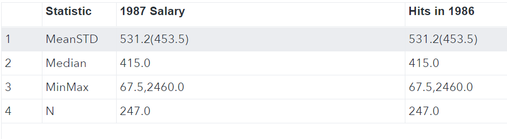## keeping the main view of data

 STATISTIC 1987 Salary Hits in 1986 N XX XX Mean (Std) XX.X (XX.XX) XX.X (XX.XX) Median XX.X XX.X Min, Max XX, XX XX, XX

when i merge 2 datasets together i see that the priority of Statistics has been mixed like this1 ACCEPTED SOLUTION

Accepted Solutions

## Re: keeping the main view of data

Add a sequence variable to newone and newone1 and then after the merge you have DATA DOUBLE, sort that by the sequence number.

--
Paige Miller
4 REPLIES 4

## Re: keeping the main view of data

We would need to see the code you are using.

--
Paige Miller

## Re: keeping the main view of data

Here you go

%macro cplayers(DS=,var1=,var2=);
proc freq data=&DS.;
tables &var1.*&var2.;
run;
%mend cplayers;

%cplayers(DS=players,var1=div,var2=team);
%cplayers(DS=players,var1=league,var2=position);
Run;

%macro baseplay(Datab=,var1=,var2=);
proc means data=&Datab.;
Var &var1. &var2.;
output out=baseplay_1 N=N1 Mean=Mean1 Std=Std1 Median=Median1 Min=Min1 Max=Max1;
run;
%mend baseplay;
%baseplay(Datab=players,var1=salary,var2=nhits);
;
run;

data newdat;
set Baseplay_1;
N=strip(put(N1,20.1));
MeanSTD=strip(put(Mean1,20.1)) || '(' || strip(put(Std1,20.1))|| ')';
Median=strip(put(Median1,20.1));
MinMax=strip(put(Min1,20.1))|| ',' || strip(put(Max1,20.1));
run;

proc transpose data = newdat out=newdat1;
Var N MeanSTD Median MinMax;
run;

%let n= 1987 Salary;
data newone (rename =(_Name_=Statistic Col1="&n"n));
set newdat1;
run;

%let m= Hits in 1986;
data newone1(rename=(_Name_=Statistic Col1="&m"n));
set newdat1;
run;

proc sort data=newone;
by Statistic;
run;

proc sort data=newone1;
by descending Statistic;
run;

data double;
merge newone newone1;
;
run;

## Re: keeping the main view of data

Add a sequence variable to newone and newone1 and then after the merge you have DATA DOUBLE, sort that by the sequence number.

--
Paige Miller

## Re: keeping the main view of data

Thanks Genius.

Discussion stats
• 4 replies
• 219 views
• 0 likes
• 2 in conversation